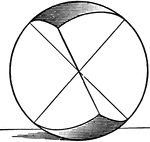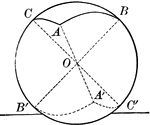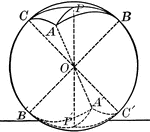### Symmetric Spherical Triangles

Illustration of symmetrical spherical triangles.### Symmetric Spherical Triangles

Illustration of symmetrical spherical triangles.### Symmetric Spherical Triangles

Illustration of symmetrical spherical triangles.